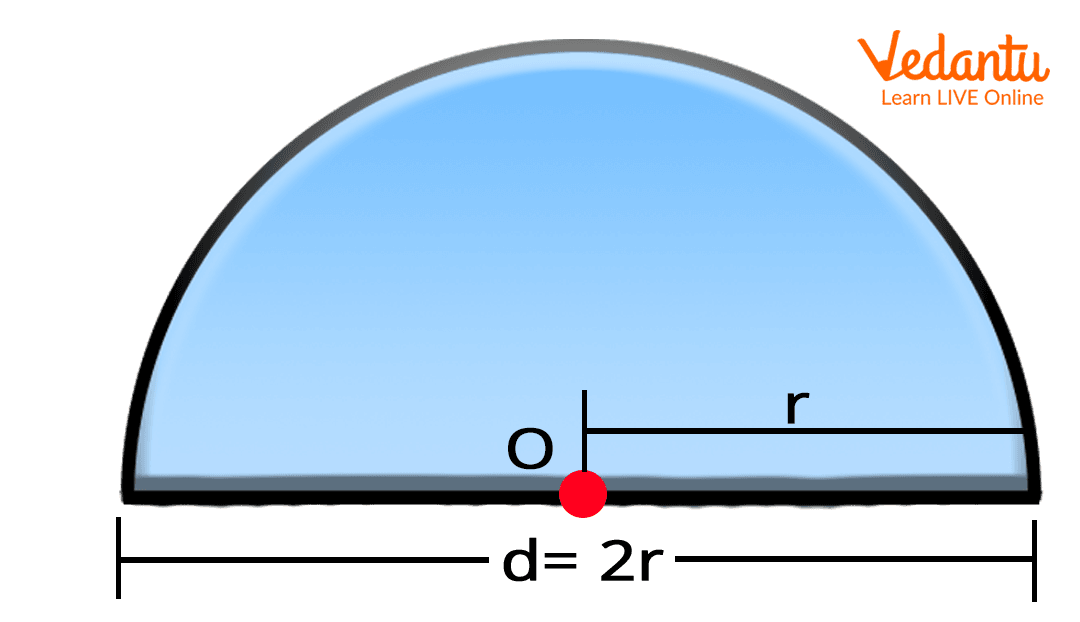Courses
Courses for Kids
Free study material
Free LIVE classes
More

# Half Circle – Definition, Properties, Formula, and ExamplesLIVE
Join Vedantu’s FREE Mastercalss

## What Does a Half Circle Look Like?

You might have observed that your forehead forms a half circle, or if you look at the sky at night, the moon appears like half of the circle. What is this half circle? Well, it is a semi-circle. You can form this shape by taking a circle and cutting it into two halves, where each half has the same half of the area as that of the original circle.

There are various parameters to express the property of a half circle; these parameters include the diameter, area, and perimeter that can be calculated using semi-circle formulas that we will discuss here.

Besides this, the diameter of a circle divides the circle into two equal semicircles. The area of any semicircle is half of the area of a circle. Now, let us understand the semicircle formulas using solved examples.

## Formula of a Half Circle

The below image shows the properties of a semicircle and that’s how we will determine the perimeter (circumference) and the area of a semicircle:A Half circle

## Area of a Semicircle Formula

From the above text, we got to know that a semicircle is half a circle, which means that the semicircle area is half of the area of a circle. So, the area of a circle is πR2 where R is the radius of the circle. The area of a semi-circle refers to the region or inner space of the semi-circle.

Now, we know that the radius of a circle is ‘r’, and the area of a circle is:

A = πr2

Now, the area of semicircle becomes:

A = r2)/2

where r = R is the radius of a semicircle and π(pi) is $\frac{22}{7}$ or 3.142 approximately.

## Perimeter of a Semicircle Formula

We use the perimeter of a semicircle formula to calculate the perimeter of a semicircle. For this, we must know either the diameter or radius of a circle along with the length of the arc.

Now, to evaluate the length of the arc of the semicircle, we must calculate the circumference of a circle, which is as follows:

The circumference of a circle is C = πd or C = 2πr.

Further, using the value of C, we can frame the formula for the perimeter of a semicircle which is equal to the sum of half of the circumference of the circle and diameter of a circle.

The perimeter of a semicircle formula is given by,

=(πR + 2R) or R(π + 2) units.

Here,

R = radius of a semicircle

d = Diameter of a semicircle.

## Circumference of a Semicircle Formula

The circumference of a semicircle is the length of the arc around the semicircle. It is half of the circumference of a circle.

Please note that the difference between the circumference and perimeter of a semicircle is that the circumference is only the length of the arc which is the curved portion on the boundary, while the perimeter of the semicircle includes circumference and diameter both.

Hence, the formula to find the circumference of a semicircle is = πR units.

Here,

R = radius of the semicircle

Now, let us go through the solved example for using semicircle formulas.

Solved Example on Semicircle Formulas

Example 1. Determine the area of a semicircle whose diameter is 14 cm.

Solution:  We are given with d = 14 cm, so the radius becomes $\frac{14}{2}$ cm or 7 cm.

Now, putting the value in the formula, we get:

A = $\frac{1}{2}$ r2)

= $\frac{1}{2}$ (π x 72) = 3.14 ($\frac{1}{2}$ x 72) = 3.14 x $\frac{49}{2}$

On solving, we get the area of the semicircle as 76.93 cm2.

Example 2. Calculate the circumference of a semi-circle whose diameter is 18 units.

Solution: We know the circumference of a semicircle of radius r. Now, putting these values, we have:

C= πr =  3.14 x $\frac{18}{2}$

We get the circumference as 28.26 cm.

Example 3. We are given the radius of a semicircle as 14 units. Now, using the semicircle formula, find its perimeter.

Solution: We have a radius of semicircle as 14 units.

Now, using the perimeter of a semicircle formula, we have πr + d = πr + 2r

= (14 x (22/7) + 28) units

= (44 + 28) units

= 72 units

The perimeter of the given semicircle is 72 units.

So, we got to know that the circumference of a circle is r and the perimeter of a semicircle is (πr + d). We also got to know how to use the semicircle formula in our questions.

Last updated date: 28th Sep 2023
Total views: 138.6k
Views today: 2.38k

## FAQs on Half Circle – Definition, Properties, Formula, and Examples

1. How many sides does a semicircle have?

A semicircle has one straight side, one curved side, and two vertices. The curved side and the straight side meet at the two ends of the straight side, also, two angles at the ends of the straight side and forming 90-degrees with the tangents to the curved side.

2. What is the formula for area and perimeter of a semi circle?

The area of a circle is given by the formula:

Area = πr2

Therefore, area of a semicircle = (πr2)/2

The formula to calculate perimeter of a semi circle = πr + d.